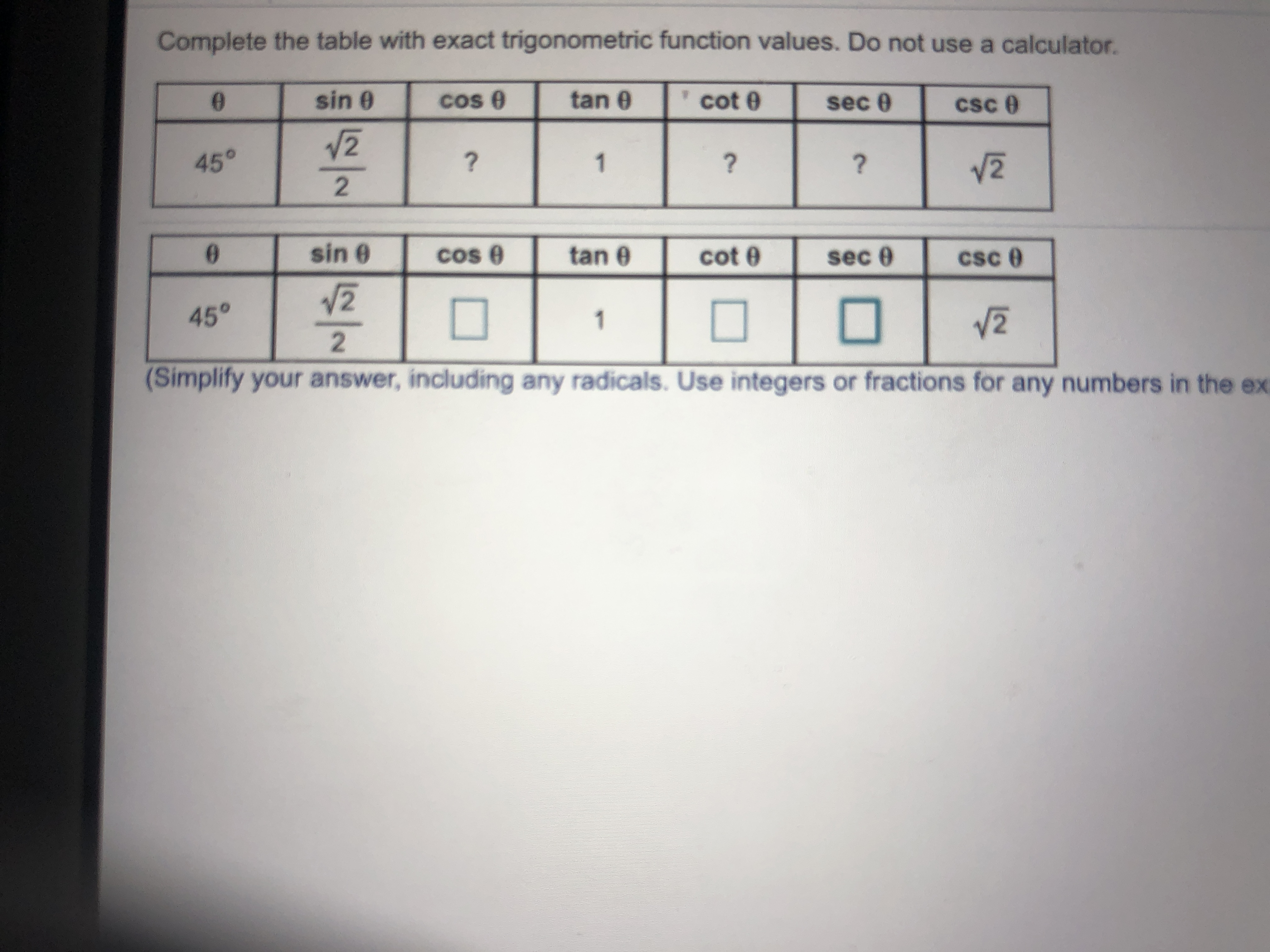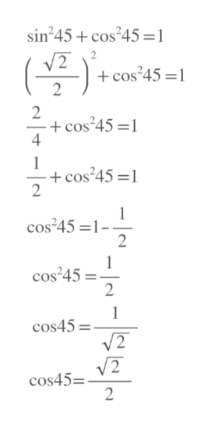Complete the table with exact trigonometric function values. Do not use a calculator.0sin 0COstan 0cot esec 0cSc245?122sinCOs etan ecotsec45212(Simplify your answer, including any radicals. Use integers or fractions for any numbers in the ex

Questionhelp_outlineImage TranscriptioncloseComplete the table with exact trigonometric function values. Do not use a calculator. 0 sin 0 COs tan 0 cot e sec 0 cSc 2 45 ? 1 2 2 sin COs e tan e cot sec 45 2 1 2 (Simplify your answer, including any radicals. Use integers or fractions for any numbers in the ex fullscreen
Step 1

We use Pythagorean identity sin^2 x+ cos^2 x=1

with x=45

From there we find cos45, and since cos 45 is in first quadrant , it will be positive.

cos 45= sqrt(2)/2help_outlineImage Transcriptionclosesin 45+cos245 =1 -cos 45 1 2 cos245 2 + cos245 =1 4 1 + cos245 =1 2 cos 45 1 2 cos45 2 1 cos45= V2 2 cos45= 2 .- fullscreen
Step 2

We use inverse trigonometric formula to f...

Want to see the full answer?

See Solution

Want to see this answer and more?

Our solutions are written by experts, many with advanced degrees, and available 24/7

See Solution
Tagged in

Other